# ILP Part 35 — Approximation

This is the thirty fifth part of the ILP series. For your convenience you can find other parts in the table of contents in Part 1 – Boolean algebra

Last time we saw how to implement Special ordered sets in ILP. Today we are going to use this knowledge to implement piecewise linear approximation in one dimension and in two dimensions. Let’s start.

# Idea

Let’s take simple non-linear function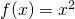. We already know how to multiply numbers in ILP but representing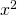in this way might be computationally intensive and hence we would like to avoid this kind of formulation. However, if we don’t need to be 100% precise it might be better to approximate this function if we know the possible range of. Let’s assume that we have these points: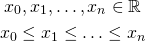we are now able to calculate the following values: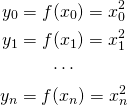So for every known argument we simply calculate the function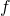. If all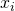are integers this looks very trivial, however, ifare reals andis a bit more complicated then precalculating the function value might greatly simplify the ILP model for our problem.

Having all these points, we declare variable representing actual argument: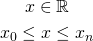So we defineas real number in range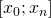, which we will use as an argument of function. However, instead of simply calculatingwe will approximate it using linear functions in ranges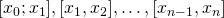.

# 1D Approximation

We will use the following trick: for all linear functions we define “weights” of vertices and use them to approximate function. They are defined in the following manner: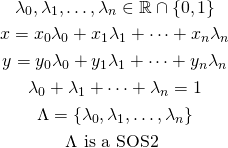Introduced lambda variables guarantee thatwill lie in one of the segments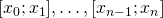thanks to SOS2 constraints. Without this stipulation would allow thevariable to “lie” on other segments, probably ranging over multiplevariables. The same goes forvariable — by using lambda variables we are able to approximatecorrectly.

Let’s consider an example: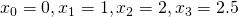with functionand values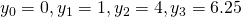. We have the following relation: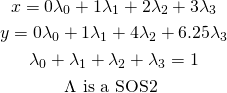And now imagine that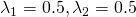, we get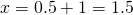and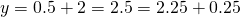which is quite a good approximation. However, if it happens thatis on a grid (which we can force by making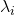binary variables), we get exact solution.

Our approximated value is represented by variableso in the remaining part of our ILP model we usein place of.

# 2D Approximation

Approximation in two dimensions goes almost exactly the same. We have the function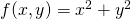and the following points: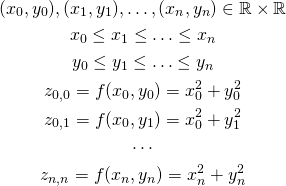We introduce the following variables: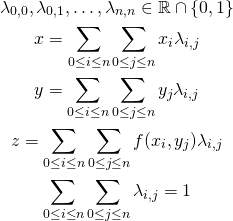We now need to add constraint similar to SOS2 for 1D approximation. In that case the constraint simply meant “at most two neighbouring variables are allowed to be non-zero”. Now we need to add constraint “at most four neighbouring variables are allowed to be non-zero” which is simply a generalization of SOS2 (bonus chatter: you might guess how to approximate 3D function). We introduce the following variables: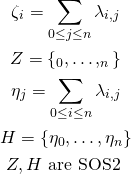If we allow lambdas to be real numbers (e.g., we don’t requireandto lay on a grid), we need to make sure that non-zero lambda variables lay on a triangle. We can do that by introducing another SOS2 set: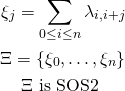# Summary

We know how to approximate 1D and 2D functions using special ordered sets of type 2. By using this approach we can greatly reduce size of ILP models which might significantly decrease solving time.